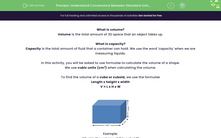# Understand Conversions Between Standard Units of Volume

In this worksheet, students will use their knowledge of calculating the volume of a cube and cuboid to solve various worded problems.Key stage:  KS 2

Curriculum topic:   Measurement

Curriculum subtopic:   Convert Between Standard Units

Difficulty level:#### Worksheet Overview

What is volume?

Volume is the total amount of 3D space that an object takes up.

What is capacity?

Capacity is the total amount of fluid that a container can hold. We use the word 'capacity' when we are measuring liquids.

In this activity, you will be asked to use formulae to calculate the volume of a shape.

We use cubic units (cm³) when calculating the volume.

To find the volume of a cube or cuboid, we use the formulae

Length x height x width

V = L x H x WExample:

What is the volume of this cuboid?V = L x H x W

V = 5 x 3 x 9

V = 15 x 9 = 135 cm³

### What is EdPlace?

We're your National Curriculum aligned online education content provider helping each child succeed in English, maths and science from year 1 to GCSE. With an EdPlace account you’ll be able to track and measure progress, helping each child achieve their best. We build confidence and attainment by personalising each child’s learning at a level that suits them.

Get started